# Adaptive system and network management (Leuven, Torino, Syllogic)

 Application domain: Adaptive system and network management Source: Syllogic for system managment, Cabletron for Network Management, Torino for additional system management and access control data Dataset size: network management (Cabletron): 5269209 bytes, system management (Leuven): 71844 bytes, system management (Syllogic+Torino): 1102111 bytes, intrusion detection (Torino): about 2 Mbytes Systems Used: C4.5, Regal, ReliC Pointers: http://www.di.unito.it/~mluser/

## System Management

Last year's experiments started by using a set of 1000 examples provided to us by Luc Dehaspe in his experiments with Claudien, and gathered by using the scripts provided by Syllogic. This year, new data acquired at Syllogic were available.

Each fact is made of 14 parameters:

number of users logged to the system, number of non root users logged on, number of processes on the system, number of non root processes, number of defunct processes, number of full file systems, number of full i-nodes tables, quantity of free space in the tmp file system (Kilobytes), percentage of paging space used, system load, percentage of cpu time used by the users, percentage of cpu time used by the system, percentage of cpu time the system was idle, percentage of cpu time used in I/O operations.

REGAL was requested to find a set of disjuncts covering the 100 facts stating that the sysload is high (sysload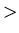3, see below). To find a disjunct covering a fact at time X, REGAL was allowed to use facts at time X-20, X-40, X-60 and X-80 (numbers are seconds). Thus, each disjunct involves a prediction gap of 20 seconds from NOW. We used a set of binary predicates, for each parameter in each fact. Each parameter was discretized defining an allowed interval and step.

Moreover, this year we added to the above environment a binary predicate, sysgrow(Y,Z), which is true iff:

(Z - W)/3 + (Y - Z)2/3 + (K-Y)0

and false otherwise. Where W is the SYSTEM_LOAD value at time X - 80 seconds, Z is SYSTEM_LOAD at time X - 60 seconds, Y is SYSTEM_LOAD at time X - 40 seconds and K is SYSTEM_LOAD at time X - 20 seconds1. The intuitive meaning of this predicate is to take care of the possible fluctuations of the system load within the four tuples of each example, giving more importance to the fluctuations closer to NOW. With this new predicate we got the following average outcomes over the three runs with last year's data:

percentage of covered examples of class low'' in the test set: 1.5%
percentage of covered examples of class average'' in the test set: 15.93%
percentage of covered examples of class high'' in the test set: 79.56%
accuracy = 89.66%

That is, the new predicate improves the predictive power of the learned rules of more than 10%. Since the obtained results were promising, we went on with the experiments using examples gathered during two days on three different computers at Syllogic. We report here about only one of them, an IBM RS/6000 running AIX 3.2.5.

Snapshots were taken each 60 seconds. The system load was classified as low'' for SYSTEM_LOAD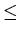0.86, average'' for 0.86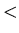SYSTEM_LOAD1.33 and high'' for SYSTEM_LOAD1.33. there are about 25% of examples of class high'' out of about 750 examples. Since the parameter values recorded at each snapshot were quite different from those of the previous experiments. discretization values and intervals had to be redefined accordingly. In particular, we see that the gap between a low'' and a high'' SYSTEM_LOAD is less than 0.5. As a consequence, the discretization of SYSTEM_LOAD used above (ten intervals of 0.5 each one) would be useless. Hence, we used a discretization in 50 intervals with a step of 0.2.

The average outcomes over the three runs as in the previous experiments is as follows:

percentage of covered examples of class low'' in the test set: 4.4%
percentage of covered examples of class average'' in the test set: 13.19%
percentage of covered examples of class high'' in the test set: 78.26%
accuracy = 86.8%

We see that prediction power and accuracy still remain quite good even for rules that must foresee the system load evolution after 60 seconds (that is, three times later than in the previous experiments) and the gap among the three classes (low, average and high) are smaller.

## Network Management

The experiments were conducted using a very large dataset given to us by L. Lewis, a researcher of Cabletron Corporation. Data were collected as a result of collaboration with Syllogic. These data were collected monitoring the Cabletron network over a period of 18 weeks in 10 minute increments. During the 18 weeks period, the administrators noted that when the load on subnet 5 was greater than 35%, the user working on that network began to complain because it was too slow. The administrator made a process that warned him whenever the load on subnet 5 was closer to 35%, in order to prevent the reaching of that threshold. The datamining task is to discover what is the causing factor of the overloading on subnet 5 for improving the entire network performances; another goal is to find more complex relationships between subnetworks, for preventing overloading whithout simply looking at the value of load.

The data were formatted into the 57 comlumn table with 16849 rows. Each row represents a snapshot of the state of the network system. The provided information about this state consists of the following fields:

• Record - the number of the record.
• Day - values in [1..7]. Sunday is 1, Monday is 2, etc.
• Time - values in [1..144]. 12:00AM is 1, 12:10AM is 2, etc.
• Load(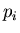) with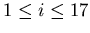- the measure of the percentage of bandwith utilization of port; note that ports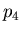and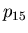were not monitored.
• Max_Load - the value of the highest load during that time increment.
• Max_Load_pt - the symbolic value of the port that had the highest load value for that time increment.
• Pkts() with- the measure of the rate at which packets are moving through the router per minute during the 10 minutes period for port(exceptand).
• Max_Pkts - the value of the highest packet rate during that time increment.
• Max_Pkts_pt - the symbolic value of the port that had the highest packet rate for that time increment.
• Coll() with- the measure of the number of packets during the interval of time that has been sent through the port(exceptand) over the link but have collided with other packets.
• Max_Coll - the value of the highest collision value during that time increment.
• Max_Coll_pt - the symbolic value of the port that had the highest collision value for that time increment.

The dataset has been distributed with this problem to solve: try to find a predictor for the value of load on subnet 5 exceeding 35%. We used three different machine learning systems: C4.5, Regal and ReliC. In the experiments, Max_Load, Max_Load_pt, Max_Pkts, Max_Pkts_ps, Max_Coll and Max_Coll_pt were not used; in fact, it is very easy to learn a rule as  if Max_Load_pt is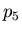and Max_Load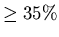then Load()''. Rules like this are obvious, useless and, sometimes, can falsify the searching in the hypothesis space. These are the description of the experiments done:

Exp-1:
The 16849 slots in the dataset are used as examples, marking as positive the cases with Load() higher than or equal 35% and as negative the other examples. Attributes are simply the fields of records in the datasets, with the exclusion of direct information on subnet 5, i.e. the attributes Load(), Pkts() and Coll() were ignored. We ran algorithms using 25% of the data for learning, and 75% for testing, with three different splittings.
Exp-2:
Each example is formed by 4 consecutive records in the dataset, and it is classified as positive (negative) if Load()(Load()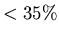) in the fifth record in the sequence; the goal is to predict, by the observation of four subsequent cases, if in the next 10 minutes Load() will overcome the given threshold. The number of the attributes are multiplied by 4 w.r.t. those used in Exp-1; more precisely we have the following: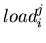-
with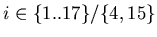and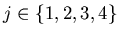- has the value of Load() for snapshot number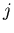in the sequence;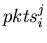-
withand- has the value of Pkts() for snapshot numberin the sequence;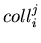-
withand- has the value of Coll() for snapshot numberin the sequence;
We ran algorithms using 25% of the data for learning, and 75% for testing, with three different splittings.
Exp-3:
Same as Exp-3, but the following relations are used instead of the attributes described above: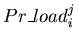-
withand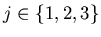- is true if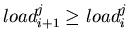and false otherwise.
Exp-4:
Same as Exp-2, with the adding of predicatesused in Exp-3;
Exp-5:
Same as Exp-4, with the adding of following predicates: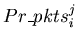-
withand- is true if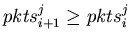and false otherwise;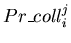-
withand- is true ifand false otherwise.
Exp-6:
Same as Exp-5, inverting training and testing sets i.e. 75% of data for training and 25% for testing.
Exp-7:
Same as Exp-2, inverting training and testing sets.

The results, for each experiment, can be seen at table below2. In the table, the averages on the three runs for accuracy, errors over positive examples and errors over negative examples, are reported for each method. Reported data are about classification of testing cases. In the case of ReliC and C4.5, only pruned tree results are given. Moreover, observe that the number of positive examples is very small (200) w.r.t. the number of the negative. This doesn't affect learning for Regal, but could change things for algortihms based on decision trees; we solved this problem duplicating the number of positive examples for the ratio number_of_negatives/number_of_positives for C4.5, and adjusting the weights for ReliC, giving the ratio above as value for class +' and value 1 for class -'.

Table: Results
 C4.5 Regal ReliC Exp. Acc. - Err + Err Acc. - Err + Err Acc. - Err + Err Exp-1 98.4% 1.1% 60.94% 95.78% 3.78% 50.81% 98.4% 1.1% 60.94% Exp-2 / / / / / / 99% 4.96% 53.6% Exp-3 / / / 98% 1.77% 69.47% / / / Exp-4 / / / 98.6% 1.04% 65.57% 98.9% 0.68% 47.2% Exp-5 / / / / / / 98.9% 0.64% 45.87% Exp-6 / / / / / / 98.27% 1.07% 37.88% Exp-7 98.23% 1.19% 34.1% / / / 98.23% 1.19% 34.1%

## Bibliography

1. F. Bergadano, D. Gunetti, F. Neri, and G. Ruffo. ILP data analysis in adaptive system and network management. Deliverable TO1b, ILP-II (second year), 1997.
2. F. Bergadano and G. Ruffo. Preliminary report on the relic learning system. Deliverable TO1a, ILP-II (second year), 1997.
3. F. Neri and L. Saitta. Exploring the power of genetic search in learning symbolic classifiers. IEEE Trans. on Pattern Analysis and Machine Intelligence, 1997.

... seconds1
It is worth noting that, since we want a prediction gap of 20 seconds, here NOW is considered to be the tuple corresponding to X - 20.
... below2
In the table there is a lack of information: in fact, some experiments were difficult to do with a particular method, if not impossible: Regal is too slow for Exp-6 and Exp-7, C4.5 is not addicted for Exp-3, Exp-4 and Exp-5, because it cannot manage relational predicates.

back to index